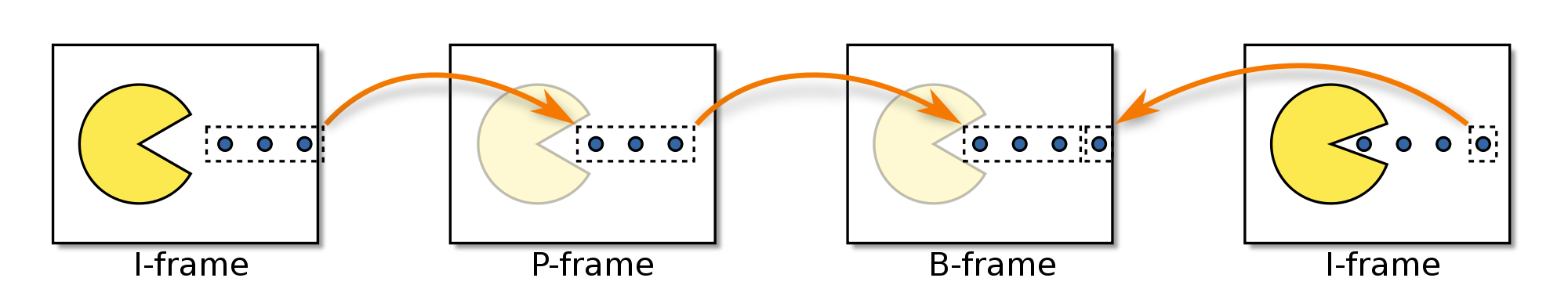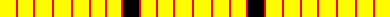### One Frame at a Time

So, we’re entering into the final weekend before the soft pencil’s down for GSoC. It’s been a very busy couple of weeks since university here in the US started 3 weeks ago. So I’ve been juggling homework, labs, and working on this. But you’re not here to for that, so let’s launch into the actual post:

Animations in-game come in two formats: AVI and a custom format called RLF. AVI is simple because I can use the ScummVM AVI decoder. But I had to reverse engineer the RLF file format so it can be played as a video or frame by frame.

Before I go into the format of the file, I want to explain the general schema of animations, or more specifically, video frame compression techniques. (For another reference, the article here is pretty good) The first frame of an animation has to include every pixel,aka, no compression. These frames are called I-frames or key frames. For the next frame, we could store every pixel, but that seems kind of wasteful. Instead, we store only the pixels that changed between the last frame and this frame. These are called P-frames. Optionally, a frame can also store the pixels that changed between the next frame and this frame. These are called B-frames. This allows animations to be played forwards or backwards. With P-frames, we can only go forwards. In order to seek within an animation, we have to find the closest I-frame, then add P/B-frames until we’re at the frame we want. To make this less painful for long animations, video encoders insert I-frames every 120 frames or so. (About 1 I-frame every 5 seconds. Assuming 24 fps, 24 * 5 = 120).RLF files only use I-frames and P-frames. If they need to go backwards, the whole animation is encoded both forwards and backwards. For example:

0, 1, 2, 3, 4, 5, 5, 4, 3, 2, 1, 0

It seems pretty wasteful in my opinion, but that’s what they do.

The RLF file starts off with a header to describe the information it contains:

bool RlfAnimation::readHeader() {
if (_file.readUint32BE() != MKTAG('F', 'E', 'L', 'R')) {
return false;
}

_frameCount = _file.readUint32LE();  // Frame count

// Since we don't need any of the data, we can just seek right to the
// entries we need rather than read in all the individual entries.
_file.seek(136, SEEK_CUR);

//_file.seek(0x18, SEEK_CUR);    // VRLE
//_file.seek(0x18, SEEK_CUR);    // HRLE
//_file.seek(0x18, SEEK_CUR);    // HKEY

_frameTime = _file.readUint32LE() / 10;  // Frame time in microseconds

return true;
}


The magic number FELR refers to the run-length encoding used in the file. I’ll explain the specifics later on. I’m kind of curious what all the extra information in the header is used for, so if you guys have any ideas, I’m all ears. The useful information is pretty self-explanatory.

After the header is the actual frame data. Each frame also has a header.

RlfAnimation::Frame RlfAnimation::readNextFrame() {
RlfAnimation::Frame frame;

uint32 size = _file.readUint32LE();          // Size
uint32 type = _file.readUint32BE();          // Either ELHD or ELRH
uint32 headerSize = _file.readUint32LE();    // Offset from the beginning of this frame to the frame data. Should always be 28

frame.encodedData = new int8[frame.encodedSize];

if (type == MKTAG('E', 'L', 'H', 'D')) {
} else if (type == MKTAG('E', 'L', 'R', 'H')) {
frame.type = Simple;
} else {
warning("Frame %u doesn't have type that can be decoded", _lastFrameRead);
}

return frame;
}


If a frame is of type DHLE, it is a P-frame, if it is of type HRLE, it’s an I-frame. We hold off decoding until we actually need to render the frame. This allows for less memory use.

So now we’ve read in all our data. How do we render a frame? The simplest case is to render the next frame. Note: _currentFrameBuffer is a Graphics::Surface that stores the current frame.

const Graphics::Surface *RlfAnimation::getNextFrame() {
assert(_currentFrame + 1 < (int)_frameCount);

if (_stream) {
} else {
applyFrameToCurrent(_currentFrame + 1);
}

_currentFrame++;
return &_currentFrameBuffer;
}

} else if (_frames[frameNumber].type == Simple) {
}
}

void RlfAnimation::applyFrameToCurrent(const RlfAnimation::Frame &frame) {
} else if (frame.type == Simple) {
decodeSimpleRunLengthEncoding(frame.encodedData, (int8 *)_currentFrameBuffer.getPixels(), frame.encodedSize, _frameBufferByteSize);
}
}


The decode....() functions simultaneously decode the frame data we read in earlier, and then blit it directly on-top of the _currentFrameBuffer pixels. I’ll explain the details of each function further down.

You might be wondering what the _stream variable refers to? I’ve created the RlfAnimation class so that it can decode in two different ways: it can load all the data from the file into memory and then do all decoding/blitting from memory, or it can stream the data from file, one frame at a time. The first option allows you to seek within the animation, but it uses quite a bit of memory (roughly the size of the file). The second option uses far less memory, but you can only play the animation forwards and can not seek.

On to the decoding functions:

I-frames contain every single pixel within a frame. Again, we could store every one of these, but that would be kind of expensive. So we use a simple compression algorithm called Run Length Encoding. (There are tons of frame compression algorithms out there. This is just the one they chose to use). Consider this image:And then, let’s choose a specific line of pixels:If we were to encode each pixel we would need to store:

YYYYYYYBYYYYYYBYYYYYYY

where Y means yellow and B means black. That’s a lot of repeated yellows. Lets instead store this:

7Y1B6Y1B

The numbers represent how many of the following pixels are of the same color. So the decoder would interpret that as: render 7 yellow pixels, 1 black pixel, 6 yellow pixels, 1 black pixel, then 7 yellow pixels.

The RLF files take this idea further. Consider this line of data, where G means green, R means red:

YYYYYBGRYBYGBYYYYYY

If we use the same method as before we get:

5Y1B1G1R1Y1B1Y1G1B6Y

It’s longer than the original data! If a color doesn’t have any repetition, using encoding actually takes up more space. To counter that, the RLF files do the following:

5Y-8BGRYBYGB6Y

If the number is negative, the next N pixels are copied directly to the destination. If it’s positive, the next N pixels are filled with the color directly following the number. Here’s that algorithm in code form:

void RlfAnimation::decodeSimpleRunLengthEncoding(int8 *source, int8 *dest, uint32 sourceSize, uint32 destSize) const {
uint32 sourceOffset = 0;
uint32 destOffset = 0;

while (sourceOffset < sourceSize) {
int8 numberOfSamples = source[sourceOffset];
sourceOffset++;

// If numberOfSamples is negative, the next abs(numberOfSamples) samples should
// be copied directly from source to dest
if (numberOfSamples < 0) {
numberOfSamples = ABS(numberOfSamples);

while (numberOfSamples > 0) {
if (sourceOffset + 1 >= sourceSize) {
return;
} else if (destOffset + 1 >= destSize) {
return;
}

byte r, g, b;
_pixelFormat555.colorToRGB(READ_LE_UINT16(source + sourceOffset), r, g, b);
uint16 destColor = _pixelFormat565.RGBToColor(r, g, b);
WRITE_UINT16(dest + destOffset, destColor);

sourceOffset += 2;
destOffset += 2;
numberOfSamples--;
}

// If numberOfSamples is >= 0, copy one sample from source to the
// next (numberOfSamples + 2) dest spots
} else {
if (sourceOffset + 1 >= sourceSize) {
return;
}

byte r, g, b;
_pixelFormat555.colorToRGB(READ_LE_UINT16(source + sourceOffset), r, g, b);
uint16 sampleColor = _pixelFormat565.RGBToColor(r, g, b);
sourceOffset += 2;

numberOfSamples += 2;
while (numberOfSamples > 0) {
if (destOffset + 1 >= destSize) {
return;
}

WRITE_UINT16(dest + destOffset, sampleColor);
destOffset += 2;
numberOfSamples--;
}
}
}
}


To encode the P-frames, we use a similar method as above. Remember that P-frames are partial frames. They only include the pixels that changed from the last frame. An example pixel line could look like this, where O is a placeholder for empty space:

OOOOBRGOOYYBRGOOOOO

To encode this we do the following:

4-3BRG2-5YYBRG5

If the number read is positive, the next N pixels should be skipped. If the number is negative, the next N pixels should be copied directly to the destination. Here is that algorithm in code form:

void RlfAnimation::decodeMaskedRunLengthEncoding(int8 *source, int8 *dest, uint32 sourceSize, uint32 destSize) const {
uint32 sourceOffset = 0;
uint32 destOffset = 0;

while (sourceOffset < sourceSize) {
int8 numberOfSamples = source[sourceOffset];
sourceOffset++;

// If numberOfSamples is negative, the next abs(numberOfSamples) samples should
// be copied directly from source to dest
if (numberOfSamples < 0) {
numberOfSamples = ABS(numberOfSamples);

while (numberOfSamples > 0) {
if (sourceOffset + 1 >= sourceSize) {
return;
} else if (destOffset + 1 >= destSize) {
return;
}

byte r, g, b;
_pixelFormat555.colorToRGB(READ_LE_UINT16(source + sourceOffset), r, g, b);
uint16 destColor = _pixelFormat565.RGBToColor(r, g, b);
WRITE_UINT16(dest + destOffset, destColor);

sourceOffset += 2;
destOffset += 2;
numberOfSamples--;
}

// If numberOfSamples is >= 0, move destOffset forward ((numberOfSamples * 2) + 2)
// This function assumes the dest buffer has been memset with 0's.
} else {
if (sourceOffset + 1 >= sourceSize) {
return;
} else if (destOffset + 1 >= destSize) {
return;
}

destOffset += (numberOfSamples * 2) + 2;
}
}
}


Whew! Almost there. The last thing to talk about is frame seeking. This requires that you’re not streaming directly from disk. (Well, you could do it, but it would probably be more trouble than it was worth). As we read in the frames, we stored which frames were I-frames. So to seek to a frame, we iterate through that list of I-frames and find the I-frame closest to our destination frame. Then we use applyFrameToCurrent() to move from the I-frame to the destination frame:

void RlfAnimation::seekToFrame(int frameNumber) {
assert(!_stream);

_currentFrame = -1;
return;
}

int closestFrame = _currentFrame;
int distance = (int)frameNumber - _currentFrame;
for (Common::List<uint>::const_iterator iter = _completeFrames.begin(); iter != _completeFrames.end(); iter++) {
int newDistance = (int)frameNumber - (int)(*iter);
if (newDistance > 0 && (closestFrame == -1 || newDistance < distance)) {
closestFrame = (*iter);
distance = newDistance;
}
}

for (int i = closestFrame; i <= frameNumber; i++) {
applyFrameToCurrent(i);
}